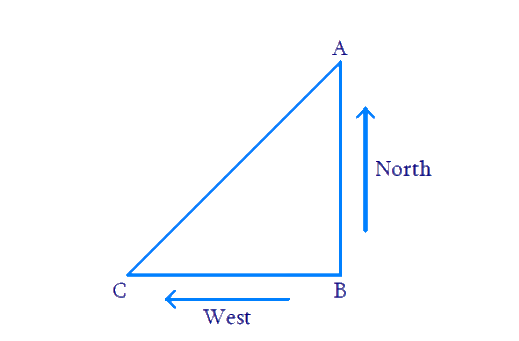# Ex.6.5 Q11 Triangles Solution - NCERT Maths Class 10

Go back to  'Ex.6.5'

## Question

An aeroplane leaves an airport and flies due north at a speed of $$1000\; \rm{}km$$ per hour. At the same time, another aeroplane leaves the same airport and flies due west at a speed of $$1200\; \rm{}km$$ per hour. How far apart will be the two planes after $$\,1\frac{1}{2}$$ hours?

DiagramVideo Solution
Triangles
Ex 6.5 | Question 11

## Text Solution

Reasoning:

We have to find the distance travelled by aeroplanes, we need to use

$\text{Distance}= \text{Speed} \times \text{Time}$

In a right triangle, the square of the hypotenuse is equal to the sum of the squares of the other two sides.

Steps:

$$AB$$ is the distance travelled by aeroplanes travelling towards north

\begin{align} A B &=1000\, \mathrm{km} / \mathrm{hr} \times 1 \frac{1}{2} \mathrm{hr} \\ &=1000 \times \frac{3}{2} \mathrm{km} \\ {AB} &=1500\, \mathrm{km} \end{align}

$$BC$$ is the distance travelled by another aeroplane travelling towards south

\begin{align} BC&=1200\,\,\text{km/hr}\times 1\frac{1}{2}\,\,\text{hr} \\ \,\,\,\,\,\,\,\,\,\,&=1200\times \frac{3}{2}\text{hr} \\ BC&=1800\,\,\text{km} \\ \end{align}

Now, In $$\Delta ABC\,\,,\,\,\angle ABC={{90}^{0}}$$

\begin{align} AC{}^{2}&=A{{B}^{2}}+B{{C}^{2}}\\ & [\text{Pythagoras }\text{theorem}] \\\\ & ={{(1500)}^{2}}+{{(1800)}^{2}} \\ & =2250000+3240000 \\ A{{C}^{2}}&=5490000 \\ AC&=\sqrt{549000} \\ \,\,\,\,\,\,\,\,\,\,\,&=300\sqrt{61}\,\,\text{km} \end{align}

The distance between two planes after  $$1\frac{1}{2}\text{hr}=300\sqrt{61}\,\,\text{km}$$

Learn from the best math teachers and top your exams

• Live one on one classroom and doubt clearing
• Practice worksheets in and after class for conceptual clarity
• Personalized curriculum to keep up with school